# Martin's Blog

## Vector extensions of abelian varieties

Posted by Martin Orr on Thursday, 17 April 2014 at 18:12

A theme of my posts on abelian varieties has been ad hoc constructions of objects which are equivalent to the (co)homology of abelian varieties together with their appropriate extra structures -- the period lattice for singular homology and the Hodge structure, the Tate module for-adic cohomology and its Galois representation. I want to do the same thing for de Rham cohomology. To prepare for this, I need to discuss vector extensions of abelian varieties -- that is extensions of abelian varieties by vector groups.

In this post I will define and classify extensions of an abelian variety by the additive group. We will conclude that, the set of isomorphism classes of such extensions, forms a vector space isomorphic to the tangent space of the dual of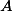. Most of this was discovered by Rosenlicht in the 1950s.

Read more...

## Endomorphisms of simple abelian varieties

Posted by Martin Orr on Thursday, 03 January 2013 at 15:51

Today I will discuss the classification of endomorphism algebras of simple abelian varieties. The endomorphism algebra of a non-simple abelian variety can easily be computed from the endomorphism algebras of its simple factors. For a simple abelian variety, its endomorphism algebra is a division algebra of finite dimension over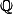. (A division algebra is a not-necessarily-commutative algebra in which every non-zero element is invertible.) As discussed last time, the endomorphism algebra also has a positive involution, the Rosati involution. There may be many Rosati involutions, coming from different polarisations of the abelian variety, but all we care about today is the existence of a positive involution. Division algebras with positive involutions were classified by Albert in the 1930s.

Read more...

## Rosati involutions

Posted by Martin Orr on Wednesday, 05 December 2012 at 16:04

I intend to return to the basic theory of abelian varieties and write write a few posts on their endomorphism algebras and associated moduli spaces. To begin with, I will discuss the Rosati involution which is an involution of the endomorphism algebra coming from a polarisation. The existence of such an involution is crucial for the classification of endomorphism algebras which I will discuss next.

Read more...

## Main steps of the proof of the period theorem

Posted by Martin Orr on Friday, 03 August 2012 at 11:06

Today I will explain how to prove the Masser-Wüstholz Period Theorem starting from the Key Proposition, an weaker existence result for abelian subvarieties of bounded degrees. The Key Proposition and the Tangent Space Lemma, which I mention briefly, are the main results proved by Masser and Wüstholz by the techniques of transcendental number theory on their way to the Period Theorem.

Recall that the Period Theorem is a bound for the degree of the smallest abelian subvariety ofwhose tangent space contains a given period. In the Key Proposition we find a subvariety of bounded degree whose tangent space satisfies the very weak condition of being inside a chosen hyperplane in. In particularneed not be in the tangent space of the subvariety. However we use the Key Proposition and induction to prove the Strong Proposition, which gives a subvariety whose tangent space does contain. Several applications of the Strong Proposition with different hyperplanes inprove the Period Theorem.

Read more...

## The matrix lemma for elliptic curves

Posted by Martin Orr on Friday, 25 May 2012 at 14:00

Letbe a principally polarised abelian variety of dimensionover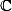. We can associate withacomplex matrix called the period matrix which roughly speaking describes a basis for the image of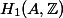in(actually it is not really the period matrix as it is only defined up to the action ofon the Siegel upper half space; we can make it nearly unique by forcing it to be in a particular fundamental domain).

The matrix lemma says that, ifis defined over a number field, then the entries of the imaginary part of the period matrix cannot be too large with respect to the height of(Faltings height or modular height).

Matrix lemma. (Masser 1987) Letbe a principally polarised abelian variety of dimensionover a number field. Letbe the period matrix forin the standard fundamental domain of the Siegel upper half space. There is a constantdepending only onsuch that all the entries ofsatisfy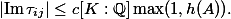Last time I used a lower bound for the lengths of non-zero periods in the proof of the isogeny theorem. This follows from the matrix lemma as we can easily relate lengths of periods and the period matrix.

Today I will prove the matrix lemma for elliptic curves. The general proof requires various facts about Siegel modular forms and also uses a funny choice of level structure due to Igusa (I do not understand why). But the basic structure of the proof is already visible in the elliptic curves case and we can be concrete about the modular forms involved, using only facts I learned in Part III.

Read more...